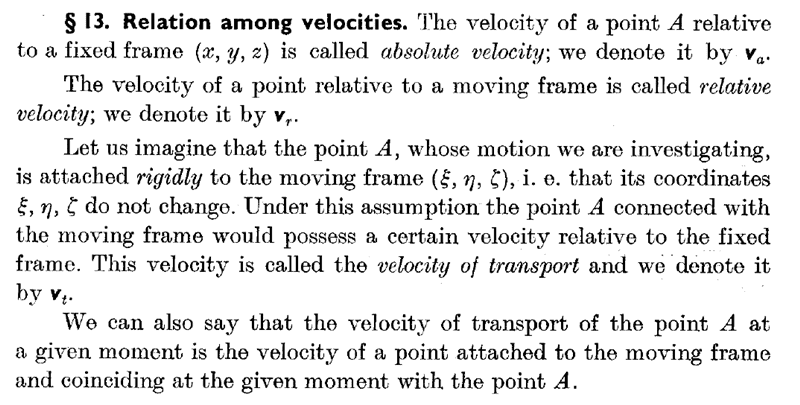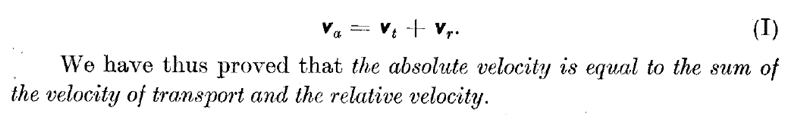# A question on terminology

• I
• wrobel
In summary, we have two coordinate frames, ##Oxyz## and ##O'x'y'z'##, where the first is fixed and the second can move or rotate. The velocity of a point relative to ##Oxyz## is given by ##\boldsymbol v=\boldsymbol v_r+\boldsymbol v_e##, where ##\boldsymbol v_r## is the velocity of the point relative to ##O'x'y'z'## and ##\boldsymbol v_e## is the velocity of the point relative to the origin of the primed frame. This term depends on the motion of the frames and the position of the point within the frame. This decomposition is useful for studying the

#### wrobel

Assume we have two coordinate frames ##Oxyz, O'x'y'z'## the first one is fixed and the second one somehow moves, perhaps rotates. Then velocity of a point relative ##Oxyz## is
##\boldsymbol v=\boldsymbol v_r+\boldsymbol v_e,## where ##\boldsymbol v_r## is point's velocity relative ##O'x'y'z'.## The question is: how is referred to as the velocity ##\boldsymbol v_e##?

Thank you

If the relative motion of the two frames is just a constant velocity at all points (uniform translation) then ##\bf v_e## is just the velocity of the origin of the primed frame relative to the origin of the unprimed frame.

If the relative motion of the two frames is not uniform translation then it becomes more complex.

andrewkirk said:
If the relative motion of the two frames is not uniform translation then it becomes more complex.
That is what I ask about. I understand the physical part of the formula, I just wonder what is the term ##\boldsymbol v_e## called in English.

Last edited:
In general, such a decomposition does not help, because ve depends on vr as soon as the frames are not in the same orientation. It is more useful to write ##\boldsymbol v=R \boldsymbol v_r+\boldsymbol v_e,## with a rotation matrix R. ve can then further be split up into components from rotation (depends on the position of the object) and linear boosts.

•QuantumQuest
mfb said:
because ve depends on vr
that is wrong. The term ##\boldsymbol v_e## has clear kinematic sense. Let ##M## stand for the point which velocity we study. Imagine that the system ##O'x'y'z'## is connected with a rigid body. And by ##P## denote the point of the rigid body that coincides with ##M## at this moment. Then ##\boldsymbol v_e## is the velocity of the point ##P## relative to ##Oxyz##. So the velocity ##\boldsymbol v_e## depends on the motion of ##O'x'y'z'## and on the position of ##M## relative ##O'x'y'z'##

mfb said:
In general, such a decomposition does not help
This decomposition is very useful and there are a lot of examples proving that

Last edited:
mfb said:
write v=Rvr+ve,\boldsymbol v=R \boldsymbol v_r+\boldsymbol v_e, with a rotation matrix R. ve
the matrices are not needed, the equality is written in vector invariant form

Consider O'=O, x=y', y=z', z=x'. Let
$$v_r = \begin{pmatrix}c\\0\\0\end{pmatrix}$$
Then
$$v = \begin{pmatrix}0\\0\\c\end{pmatrix}$$
$$v_e = \begin{pmatrix}-c\\0\\c\end{pmatrix}$$
which depends on vr.

You can not summate coordinate representations from different coordinate frames

I have found the term at last! S. Banach: Mechanics, Warszawa, 1951. Translated in English by E J Scott.
By the way, in Banach's book the moving frame can move arbitrary.wrobel said:
You can not summate coordinate representations from different coordinate frames
I can sum vectors. We are in a mathematics subforum. If you implicitly transform all vectors between different frames: fine. But then use different symbols for transformed vectors please.
Note how vr is defined in the coordinates of the fixed frame in your quoted text.

Usually this most general case of a generally accelerated reference frame occurs for the treatment of a rigid body. So in the following we think of this moving frame as fixed in the interior of a rigid body. Also all vectors are meant as ##\mathbb{R}^3## column vectors with respect to the Cartesian bases for the inertial frame (vector components ##\vec{x}##) and the body-fixed frame (vector komponents ##\vec{x}'##). Then we have
$$\vec{x}=\vec{R}+\hat{D} \vec{r}'.$$
Here ##\vec{R}## is the vector pointing from the origin of the inertial frame to an arbitrary point fixed within the body which is the origin of the body-fixed reference frame (written in components wrt. the inertial basis), ##\vec{r}'## is the vector (components wrt. the body-fixed basis) from the body-fixed origin to an arbitrary other point in the body. ##\hat{D} \in \mathrm{SO}(3)## is a rotation matrix (usually parametrized with three Euler angles). Then the components of the velocity in the inertial frame are given by
$$\vec{v}=\dot{\vec{x}}=\dot{\vec{R}} +\dot{\hat{D}} \vec{r}'. \qquad (*)$$
Here we have used that ##\vec{r}'## is fixed within the body, i.e., for an observer in the body-fixed reference frame it's a constant in time. Now we want to express this equation in terms of components wrt. the inertial basis. Now we have
$$\dot{\hat{D}} \vec{r}'=\dot{\hat{D}} \hat{D}^{-1} \vec{r}.$$
Since ##\hat{D} \in \mathrm{SO}(3)## we have ##\hat{D}^{-1}=\hat{D}^{\mathrm{T}}## and thus
$$(\dot{\hat{D}} \hat{D}^{-1})^{\mathrm{T}} = (\dot{\hat{D}} \hat{D}^{\mathrm{T}})^{\mathrm{T}} = \hat{D} \dot{\hat{D}}^{\mathrm{T}}.$$
On the other hand
$$\hat{D} \hat{D}^{\mathrm{T}} \hat{D}=1 \; \Rightarrow \; \dot{\hat{D}} \hat{D}^{\mathrm{T}} =-\hat{D} \dot{\hat{D}}^{\mathrm{T}},$$
i.e., ##\dot{\hat{D}} \hat{D}^{-1}## is an antisymmetric matrix and thus we can write
$$(\dot{\hat{D}} \hat{D}^{-1})_{jk} = \epsilon_{jlk} \omega_l.$$
Plugging this into (*) gives
$$v_j=V_{j} + \epsilon_{jlk} \omega_l r_k=V_{j} + (\vec{\omega} \times \vec{r})_j \; \Rightarrow \; \vec{v}=\vec{V}+\vec{\omega} \times \vec{r}.$$
Here ##\vec{V}=\dot{\vec{R}}## is the tranlational velocity of the body against the inertial frame, described by the velocity of the arbitrarily fixed point in the body, which is the origin of the body-fixed reference frame, and which often is convenient to be chosen as the center of mass of the body. The 2nd term describes the velocity of an arbitrary point of the body given by the relative vector ##\vec{r}## from the body-fixed origin to the point in the body because of the rotation of the body around the body-fixed origin relative to the inertial frame, and ##\vec{\omega}## is the momentary angular velocity of this rotation (which can be expressed in terms of Euler angles when needed).

•wrobel and ShayanJ
•vanhees71
That's a very nice proof too, avoiding the matrix manipulations in using directly the basis vectors :-)). It's perhaps didactically simpler than the use of SO(3) matrices.